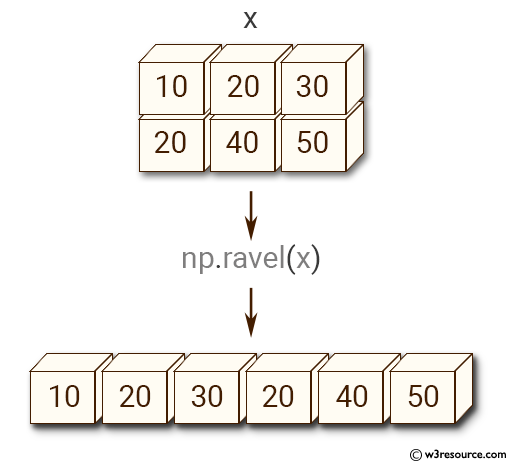﻿ NumPy: Create a contiguous flattened array - w3resource# NumPy: Create a contiguous flattened array

## NumPy: Array Object Exercise-36 with Solution

Write a NumPy program to create a contiguous flattened array.

Pictorial Presentation:Sample Solution:-

Python Code:

``````import numpy as np
x = np.array([[10, 20, 30], [20, 40, 50]])
print("Original array:")
print(x)
y = np.ravel(x)
print("New flattened array:")
print(y)
```
```

Sample Output:

```Original array:
[[10 20 30]
[20 40 50]]
New flattened array:
[10 20 30 20 40 50]
```

Python Code Editor:

Have another way to solve this solution? Contribute your code (and comments) through Disqus.

What is the difficulty level of this exercise?

Test your Python skills with w3resource's quiz

﻿

## Python: Tips of the Day

Set comprehension:

```>>> m = {x ** 2 for x in range(5)}
>>> m
{0, 1, 4, 9, 16}
```